Introduction - Coordination Chemistry

# Introduction - Coordination Chemistry Notes | Study Inorganic Chemistry - Chemistry

## Document Description: Introduction - Coordination Chemistry for Chemistry 2022 is part of Inorganic Chemistry preparation. The notes and questions for Introduction - Coordination Chemistry have been prepared according to the Chemistry exam syllabus. Information about Introduction - Coordination Chemistry covers topics like Coordination Chemistry, Coordination Compounds, Important Terms Involving Coordination Compounds, Coordination Complex, Ligands and Introduction - Coordination Chemistry Example, for Chemistry 2022 Exam. Find important definitions, questions, notes, meanings, examples, exercises and tests below for Introduction - Coordination Chemistry.

Introduction of Introduction - Coordination Chemistry in English is available as part of our Inorganic Chemistry for Chemistry & Introduction - Coordination Chemistry in Hindi for Inorganic Chemistry course. Download more important topics related with notes, lectures and mock test series for Chemistry Exam by signing up for free. Chemistry: Introduction - Coordination Chemistry Notes | Study Inorganic Chemistry - Chemistry
 Table of contents Coordination Chemistry Coordination Compounds Important Terms Involving Coordination Compounds Coordination Complex Ligands
 1 Crore+ students have signed up on EduRev. Have you?

Coordination Chemistry

Coordination chemistry is the branch of inorganic chemistry that concerns the study of coordination compounds.

Coordination Compounds

Coordination compounds are chemical compounds that consist of an array of anions or neutral molecules that are bound to a central atom via coordinate covalent bonds. Coordination compounds are also referred to as coordination complexes.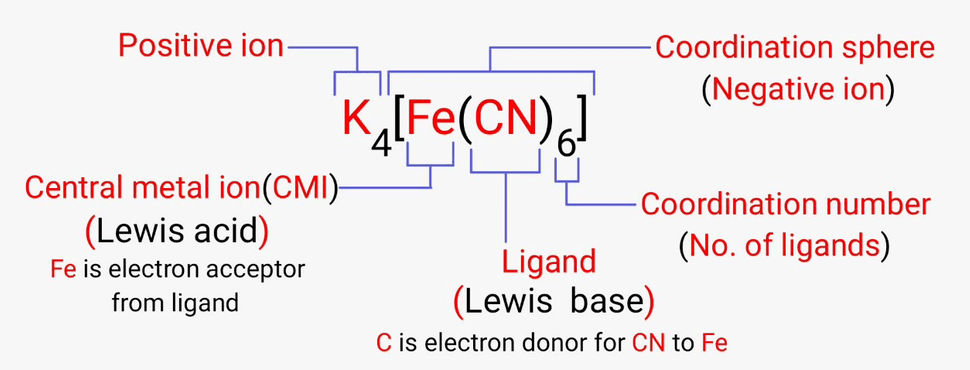• These molecules or ions that are bound to the central atom are referred to as ligands (also known as complexing agents).
• Many coordination compounds contain a metallic element as the central atom and are therefore referred to as metal complexes.
• These types of coordination complex generally consist of a transition element as the central atom. It can be noted that the central atom in these complexes is called the coordination centre.

Important Terms Involving Coordination Compounds

The definitions of some important terms in the chemistry of coordination compounds can be found below.

Coordination Entity

A chemical compound in which the central ion or atom (or the coordination centre) is bound to a set number of atoms, molecules, or ions is called a coordination entity.

Some examples of such coordination entities include [CoCl3(NH3)3], and [Fe(CN)6]4-.

Central Atoms and Central Ions

As discussed earlier, the atoms and ions to which a set number of atoms, molecules, or ions are bound are referred to as the central atoms and the central ions.

In coordination compounds, the central atoms or ions are typically Lewis Acids and can, therefore, act as electron-pair acceptors.

Coordination Number

The coordination number of the central atom in the coordination compound refers to the total number of sigma bonds through which the ligands are bound to the coordination centre.

For example, in the coordination complex given by [Ni(NH3)4]2+, the coordination number of nickel is 4.

Coordination Sphere

The non-ionizable part of a complex compound which consists of central transition metal ion surrounded by neighboring atoms or groups enclosed in square bracket.

The coordination centre, the ligands attached to the coordination centre, and the net charge of the chemical compound as a whole, form the coordination sphere when written together.

This coordination sphere is usually accompanied by a counter ion (the ionizable groups that attach to charged coordination complexes).

Example: [Co(NH3)6]C/3 – coordination sphere

Coordination Polyhedron

The geometric shape formed by the attachment of the ligands to the coordination centre is called the coordination polyhedron.

Examples of such spatial arrangements in coordination compounds include tetrahedral and square planar.

Oxidation Number

The oxidation number of the central atom can be calculated by finding the charge associated with it when all the electron pairs that are donated by the ligands are removed from it.

For example, the oxidation number of the platinum atom in the complex [PtCl6]2- is +4.

Homoleptic and Heteroleptic Complex

• When the coordination centre is bound to only one type of electron pair donating ligand group, the coordination complex is called a homoleptic complex, for example: [Cu(CN)4]3-.
• When the central atom is bound to many different types of ligands, the coordination compound in question is called a heteroleptic complex, an example for which is [Co(NH3)4Cl2]+.

Double Salts

Double salts are completely ionizable in aqueous solutions and each ion in the solution gives the corresponding confirmatory test.

Example: Potash Alum is double sulphate. It is K2SO4.Al2(SO4)3.24H2O on Ionization it gives:

K+, SO2−4 and Al+3 ions which response to the corresponding tests.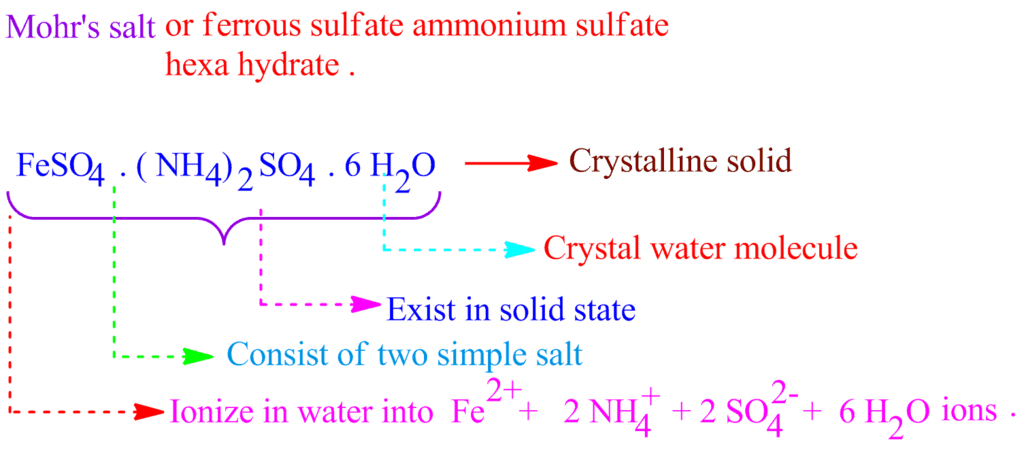Ionization of Mohr's Salt

Coordination Complex

Co-ordinate complex are incompletely ionizable in the aqueous solutions. These give a complexion which does not show complete ionization.

Example: Potassium Ferrocyanide. [K4Fe(CN)6] It ionizes to give K+ and [Fe(CN)6]−4 [ferro cyanide ions]

Types of Coordination Complexes

• Cationic complexes: In this co-ordination sphere is a cation. Example: [Co(NH3)6]Cl3
• Anionic complexes: In this co-ordination sphere is Anion. Example: K4[Fe(CH)6]
• Neutral Complexes: In this co-ordination sphere is neither cation or anion. Example: [Ni(CO)4]
• Homoleptic complex: The complex consist of a similar type of ligands. Example: K4[Fe(CN)6]
• Heteroleptic complexes: These consists of different types of ligands. Example: [Co(NH3)5Cl]SO4
• Mononuclear complexes: In this co-ordination sphere has a single transition metal ion. Example: K4[Fe(CN)6]
• Polynuclear complexes: More than one transition metal ion is present. Example:

Ligands

The atoms, molecules, or ions that are bound to the coordination centre or the central atom/ion are referred to as ligands.

• These ligands can either be a simple ion or molecule (such as Cl– or NH3) or in the form of relatively large molecules, such as ethane-1,2-diamine (NH2-CH2-CH2-NH2).
• Some common ligands, their abbreviations, formulae, and the donor atoms are given in the following table.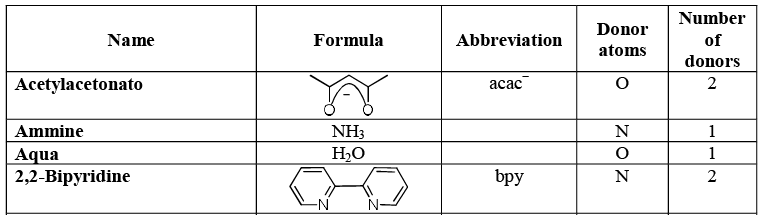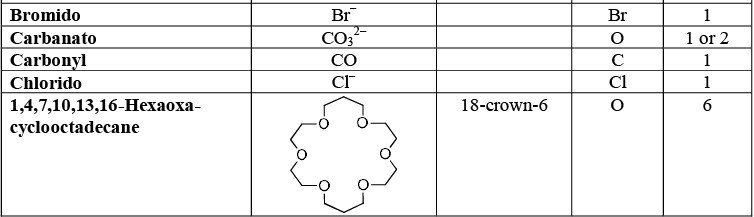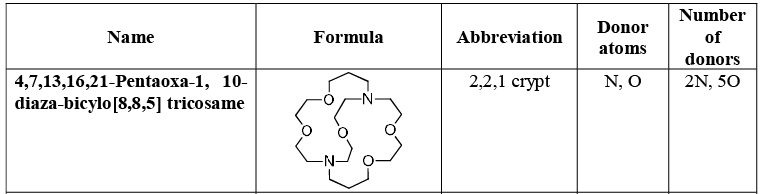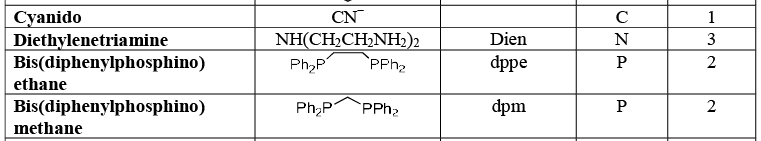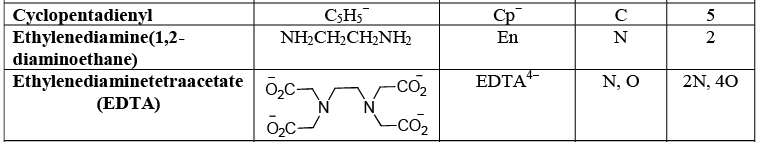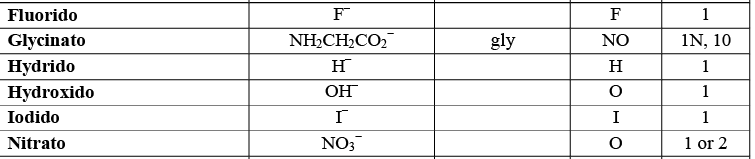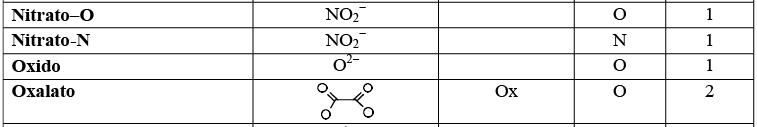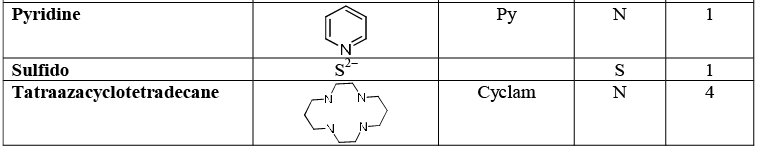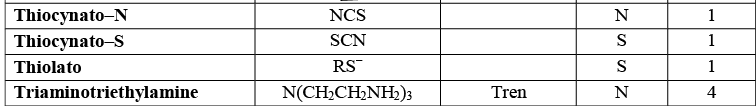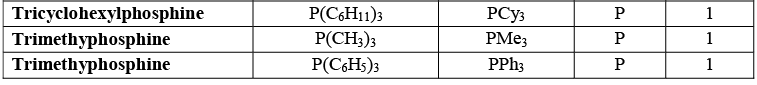The document Introduction - Coordination Chemistry Notes | Study Inorganic Chemistry - Chemistry is a part of the Chemistry Course Inorganic Chemistry.
All you need of Chemistry at this link: Chemistry

## Inorganic Chemistry

36 videos|71 docs|16 tests
 Use Code STAYHOME200 and get INR 200 additional OFF

## Inorganic Chemistry

36 videos|71 docs|16 tests

Track your progress, build streaks, highlight & save important lessons and more!

,

,

,

,

,

,

,

,

,

,

,

,

,

,

,

,

,

,

,

,

,

;